×

Search anything:

# Eigenfaces for Face recognition

#### Machine Learning (ML)Get this book -> Problems on Array: For Interviews and Competitive Programming

Reading time: 30 minutes | Coding time: 10 minutes

In this article, we have explored EigenFaces in depth and how it can be used for Face recognition and developed a Python demo using OpenCV for it.

Facial recognition techonology is used to recognise a person using an image or a video. It generally works by comparing facial features from the capured image with those already present in the database. This technology is used in entrance control, surveillance systems, smartphone unlocking etc.

We will use OpenCV for our demo. Available algorithms in the FaceRecognizer class of OpenCV are:

• Eigenfaces (createEigenFaceRecognizer())
• Fisherfaces (createFisherFaceRecognizer())
• Local Binary Patterns Histograms (createLBPHFaceRecognizer())

The basic steps involved in each of these algorithms for face recognition are :

1. Face Detection
2. Data Gathering
3. Data Comparision
4. Face Recognition

## EigenFaces

We know that every part of the face is not essential in the face recognition process. Whenever we see a person, we recognise him/her by just a few major characteristics of the face like eyes, nose, forehead. It means that we only focus on the areas of maximum variation.

Eigenfaces works on a similar principle. It takes all the training images of all the people at once and looks at them as a whole. It keeps all the required/important features and discards the rest.

The important features that the algorithm extracts are known as principle components. It also keeps a record of which features belongs to which person.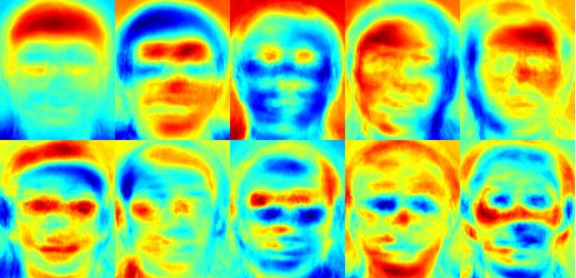The final goal of PCA is dimensionality reduction. It linearly projects original data onto a lower dimentional subspace giving the principle components maximum variance of the projected data.

Eigenfaces are images that can be added to a average (mean) face to create new facial images. Mathematically :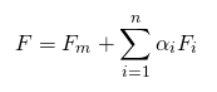• F is the new face,
• Fm is the mean face,
• Fi is an EigenFace,
• alpha_i are scalar multipliers we can choose to create new faces ( can be +ve or -ve).

The basic steps involved are:

• Find the principle components in the input image
• Compare these with those already stored in the database
• Find the best matching one
• Return the image with a label (Name/ID)

In eigenfaces illumination is also considered an important feature of the face which actually isn't and due to this some main features are discarded considering them less important. This is a major disadvantage of the eigenfaces algortihm which was later fixed by fisherfaces and LBPH algorithm.

This algorithm take in consideration the features that differentiate one individual from other. It concentrates on the features that represent all the faces of all the people.

## Main idea behind EigenFaces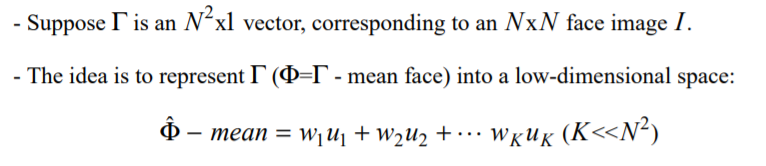## Steps in Face recognition using EigenFaces

1. Creating dataset : We need many facial images of all the individuals.
2. Alignment : Resize and reorient faces such that eyes, ears, forehead of all the faces are aligned in all the images.
3. Creating data matrix : Data matrix is created containing all images as a row vector.
4. Mean Vector : Before PCA, we need to subtract the mean vector. OpenCV does this automatically if vector if not supplied.
5. Priciple Components : These are calculated by finding the Eigenvectors of the covariance matrix. We just need to supply the data matrix to PCA in OpenCV and output comes in the form of matrix containing eigenvectors.
6. Reshape Eigenvectors to obtain EigenFaces

## Algorithm of EigenFaces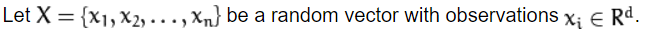1. Computing mean m_u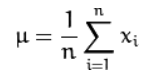1. Computing Covariance Matrix S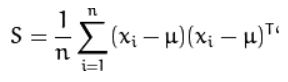1. Computing eigenvalues and eigenvectors of S: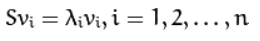1. Order the eigenvectors descending by their eigenvalue. The k principal components are the eigenvectors corresponding to the k largest eigenvalues.
The k principal components of the observed vector x are then given by: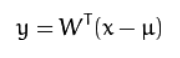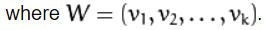1. The reconstruction from the PCA basis is given by: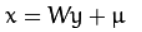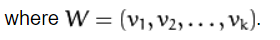Method used by Eigenfaces :

• Project all training samples into PCA subspace.
• Project the input image into the PCA subspace.
• Calculate nearest neighbor between the training images and the input image.

PCA solves the covariance matrix S = X X^{T} but sometimes it end up with a very large dimentional matrix which is not very memory efficient and takes more time to solve.

We know from linear algebra that a M x N matrix with M > N can only have N - 1 non-zero eigenvalues. So it’s possible to take the eigenvalue decomposition S = X^{T} X of size N x N instead: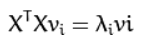and get the original eigenvectors of S = X X^{T} with a left multiplication of the data matrix: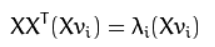## Implementation of EigenFaces for Face Recognition

We’ll use the LFW dataset in our code.

• Importing libraries, dataset, splitting data into training and testing.
``````import matplotlib.pyplot as plt
from sklearn.model_selection import train_test_split
from sklearn.datasets import fetch_lfw_people
from sklearn.metrics import classification_report
from sklearn.decomposition import PCA
from sklearn.neural_network import MLPClassifier

lfw_dataset = fetch_lfw_people(min_faces_per_person=100)

_, h, w = lfw_dataset.images.shape
X = lfw_dataset.data
y = lfw_dataset.target
target_names = lfw_dataset.target_names

# split into a training and testing set
X_train, X_test, y_train, y_test = train_test_split(X, y, test_size=0.3)
``````
• We'll now use PCA class provided by scikit-learn to perform dimentionality reduction. We'll select the no of output dimensions(components) we want to reduce down to. We'll also whiten our data.
``````# Compute a PCA
n_components = 100
pca = PCA(n_components=n_components, whiten=True).fit(X_train)

# apply PCA transformation
X_train_pca = pca.transform(X_train)
X_test_pca = pca.transform(X_test)
``````
• Now it's time to train our neural network. We'll use early stopping to avoid overfitting i.e. if our optimizer notices that our validation accuracy hasn’t increased for a certain number of epochs, then training will stop.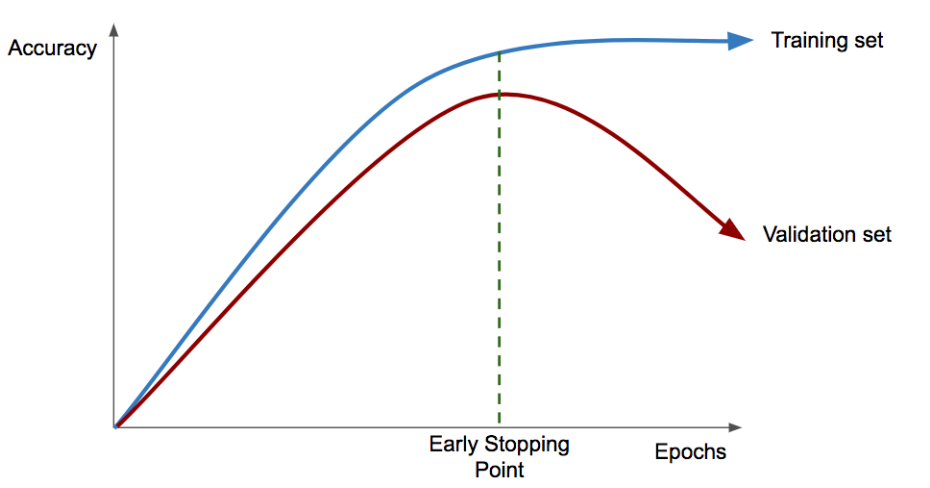``````print("Fitting the classifier to the training set")
clf = MLPClassifier(hidden_layer_sizes=(1024,), batch_size=256, verbose=True, early_stopping=True).fit(X_train_pca, y_train)
``````
• Now it's time to make a prediction, print classification report for quality check.
``````y_pred = clf.predict(X_test_pca)
print(classification_report(y_test, y_pred, target_names=target_names))
``````
• Lets test our classifier by giving it some images to classify.
``````# Visualization
def plot_gallery(images, titles, h, w, rows=3, cols=4):
plt.figure()
for i in range(rows * cols):
plt.subplot(rows, cols, i + 1)
plt.imshow(images[i].reshape((h, w)), cmap=plt.cm.gray)
plt.title(titles[i])
plt.xticks(())
plt.yticks(())

def titles(y_pred, y_test, target_names):
for i in range(y_pred.shape):
pred_name = target_names[y_pred[i]].split(' ')[-1]
true_name = target_names[y_test[i]].split(' ')[-1]
yield 'predicted: {0}\ntrue: {1}'.format(pred_name, true_name)

prediction_titles = list(titles(y_pred, y_test, target_names))
plot_gallery(X_test, prediction_titles, h, w)
``````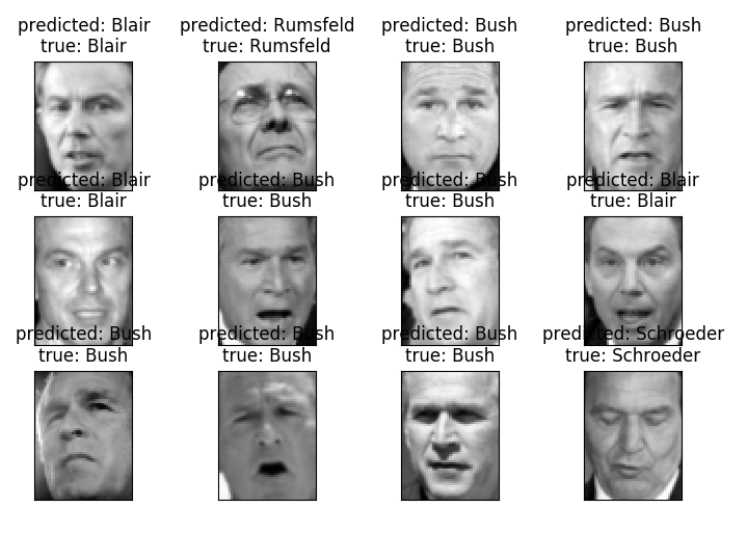We'll also visualise the eigenfaces for each image.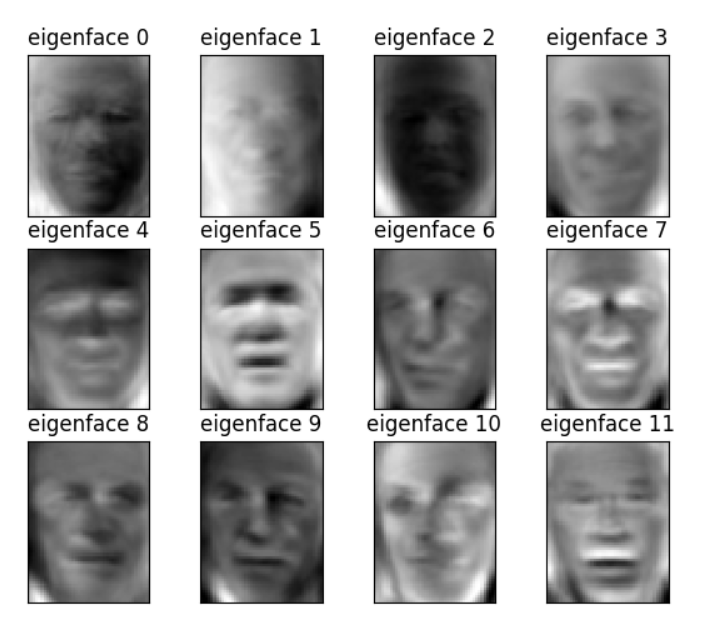## Problems with EigenFaces

• Lightning conditions impact greatly
• Recognition becomes difficult when head size changes( Scale changes )
• Orientation changes affects the recognition process
• Changes in background of the image also cause problems in recognition process

## Conclusion

• Eigenfaces are images that can be added to a mean face to create new facial images
• Here illumination is also considered an important feature of the face which actually isn't
• It uses PCA for dimentionality reduction
• After getting smaller dimensions for our images we apply the classifier that takes the reduced dimension images to produces a class label.

Face recognition is a brilliant example of merging Machine Learning with computer vision and many researches are still being done on this topic.